Open in App
Not now

# Class 12 RD Sharma Solutions – Chapter 21 Areas of Bounded Regions – Exercise 21.1 | Set 1

• Last Updated : 04 Feb, 2022

### Question 1. Using integration, find the area of region bounded between the line x = 2 and parabola y2 = 8x

Solution:

Here,

Given equations are:

x = 2       ……..(1)

y2 = 8x     ……..(2)

Here,

Equation (1) represents a line parallel to y-axis and equation (2) represents a parabola with vertex at origin and x-axis,

Here is the rough sketch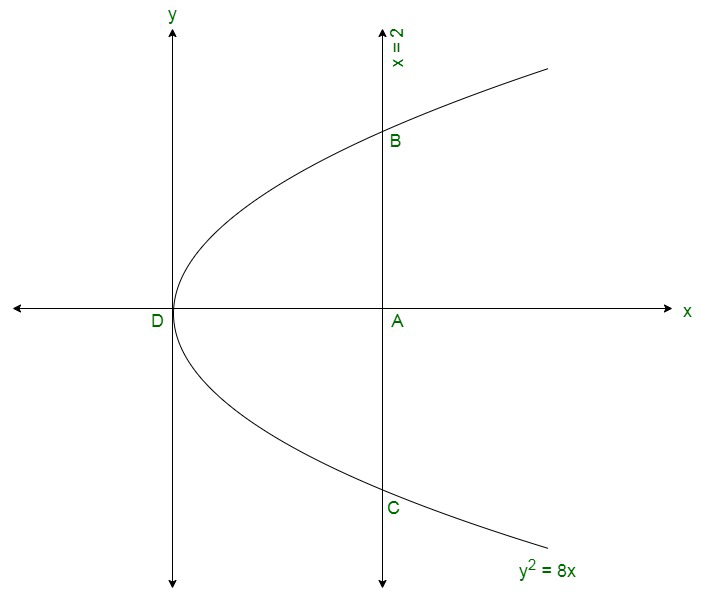We have to find the area of shaded region. We sliced it in vertical rectangle width of rectangle = △x,

Length = (y – 0) = y

Area of rectangle = y△x

This rectangle can move horizontal from x = 0 to x = 2

Required area = Shaded region OCBORequired area =square units.

### Question 2. Using integration, find the area of the region bounded by the line y – 1 = x, the x-axis and the ordinates x = -2 and x = 3.

Solution:

The find area of region bounded by x-axis the ordinates x = -2 and x = 3

y – 1 = x   ………….(1)

Equation (1) is a line that meets at axes at (0, 1) and (-1, 0)

Here, is the rough sketchRequired area is enclosed between the lines.

Required area = Region ABCA + Region ADEA### Question 3. Find the area of the region bounded by the parabola y2 = 4ax and the line x = a

Solution:

Here we have to find the area of the region that is bounded by

x = a     ………(1)

and

y2 = 4a    ………(2)

Equation (1) represents a line parallel to y-axis and equation (2) represent a parabola with vertex at origin and axis as x-axis.

Here, is the rough sketch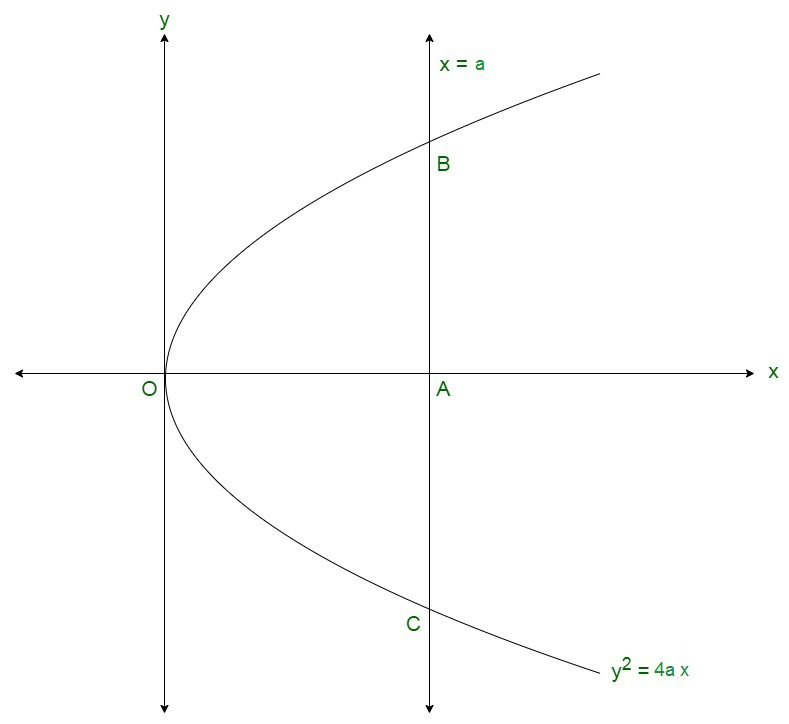Here we have to find area between the region,

Thus, slice it in rectangles of

Width = △x

Length = y – 0 = y

Area of rectangle = y△x

This assumed triangle can go from x = 0 to x = a.

Required area = Region OCBO

= 2 (Region OABO)Required area =square units

### Question 4. Find the area lying above the x-axis and under the parabola y = 4x – x2.

Solution:

We have to find here the bounded area by x-axis and parabola

y = 4x – x2

x2 – 4x +4 = -y + 4

(x – 2)2 = -(y – 4)     ……….(1)

Equation (1) represent a downward parabola with vertex (2, 4) and passing through (0, 0) and (0, 4).

Here is the rough sketch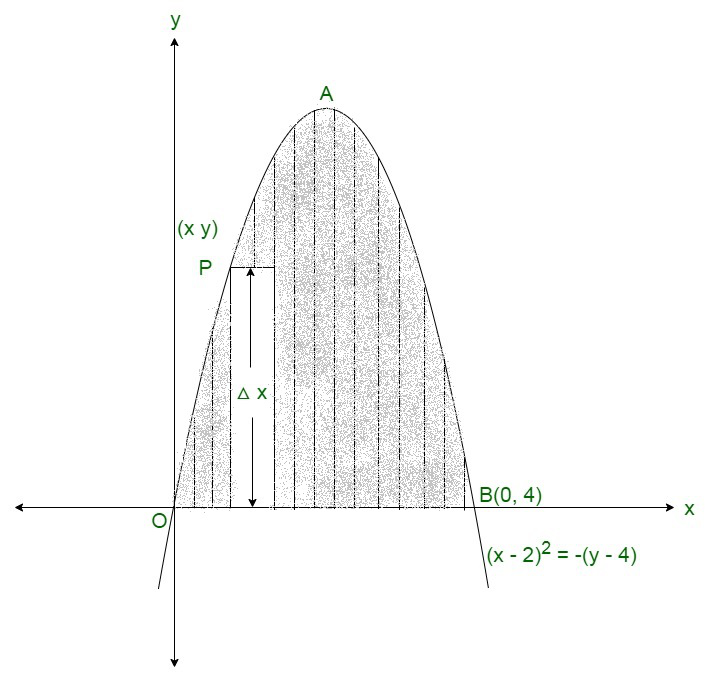Here the shaded region represents the required area.

We slice the region in approximation rectangles

Width = △x

Length = y – 0 = y

Area of rectangle = y△x

This approximation rectangle slide from x = 0 to x = a

Thus,

Required area = Region OABORequired area =square units

### Question 5. Draw a rough sketch to indicate the region bounded between the curve y2 = 4x and the line x = 3. Also, find the area of this region.

Solution:

We have to find area bounded by

y2 = 4x   ……..(1)

and

x = 3   ………..(2)

Equation (1) represents a parabola with vertex at origin and axis as x-axis and equation (2) represents a line parallel to y-axis

Here is the rough sketch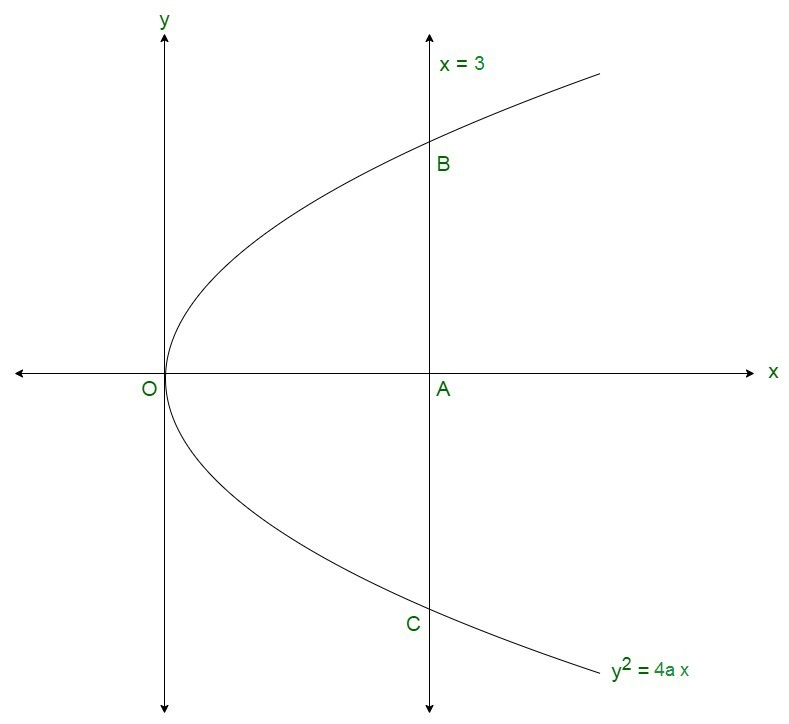Shaded region represents the required area that we have sliced in the form of a rectangle of

Width = △x

Length = y – 0 = y

This approximation rectangle slide from x = 0 to x = 3

Required area = Region OCBO

= 2(Region OABO)Required area =square units

### Question 6. Make a rough sketch of the graph of the function y = 4 – x2, 0 ≤ x ≤ 2 and determine the area enclosed by the curve, the x-axis, and the line x = 0 and x = 2.

Solution:

Here, we will find the area enclosed by

y = 4 – x2

x2 = -(y – 4)     ……….(1)

x = 0    ………(2)

x = 2     ……….(3)

Equation (1) represents a downward parabola with vertex at (0, 4) and passing through (2, 0), (-2, 0). Equation (2) represents y-axis and equation (3) represents a line parallel to y-axis.

Here’s a rough sketch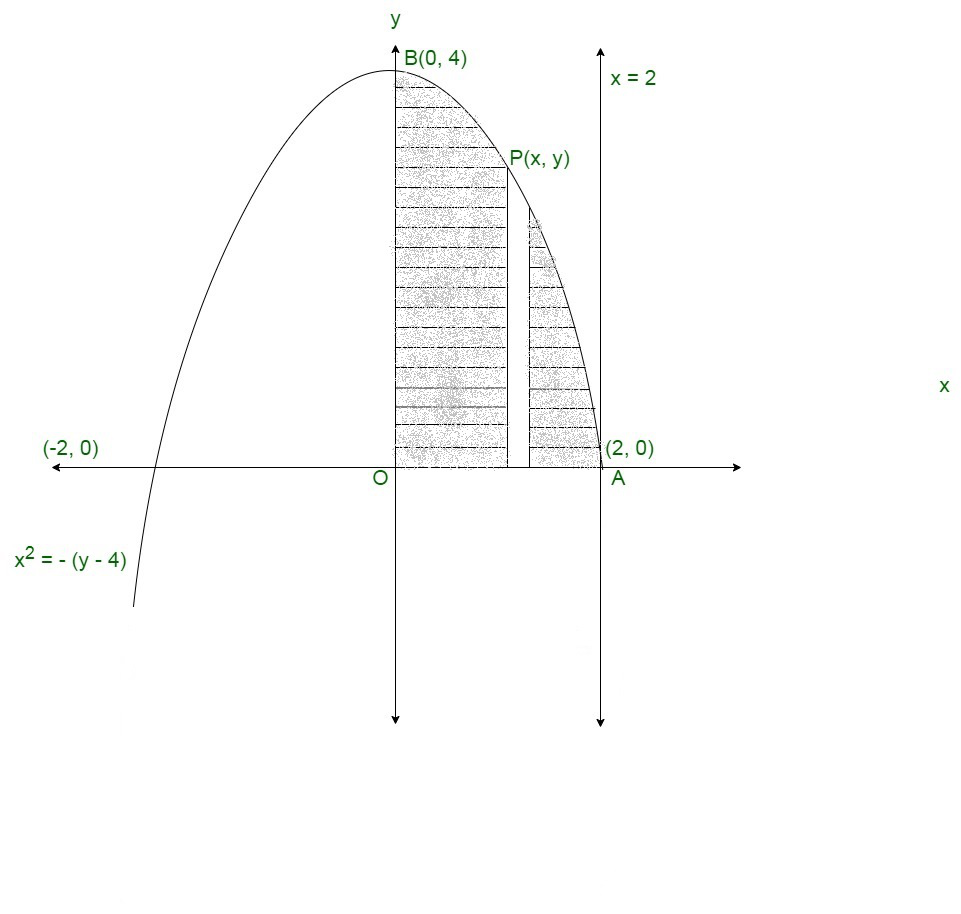Shaded region represents the required area that we have sliced in the form of a rectangle of

Width = △x

length = y – 0 = y

This approximation rectangle slide from x = 0 to x = 2

Required area = Region OABORequired area =square units.

### Question 7. Sketch the graph ofin [0, 4] and determine the area of the region enclosed by the curve, the x-axis and the line x = 0 and x = 4.

Solution:

Here, we will find the area enclosed by x-axis andy2 = x + 1  …….(1)

x = 0   ………(2)

x = 4    ………(3)

Equation (1) represents a parabola with vertex at (-1, 0) and passing through (0, 1) and (0, -1). Equation (2) represents y-axis and equation (3) represents a line parallel to y-axis passing through (4, 0).

Thus, here is the rough sketch;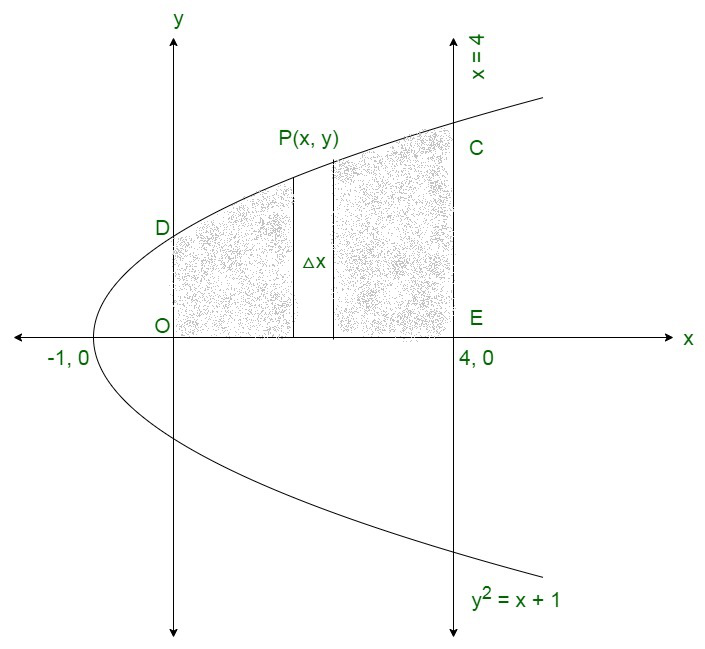Shaded region represents the required area that we have sliced in the form of a rectangle of

Width = △x

Length = y – 0 = y

Area of rectangle = y△x

This approximation rectangle slide from x = 0 to x = 4

Required area = Region OECDORequired area =square units orsquare units.

### Question 8. Find the area under the curveabove x-axis from x = 0 to x = 2. Draw a sketch of the curve also.

Solution:

Here, we will find the area enclosed by x-axis

x = 0,

x = 2    ………(1)

y2 = 6x + 4     ……….(2)

Equation (1) represents y-axis and a line parallel to y-axis passing through (2, 0). Equation (2) represents a parabola with vertex atand passes through the points (0, 2) , ( 0, -2).

Thus, here is the rough sketch;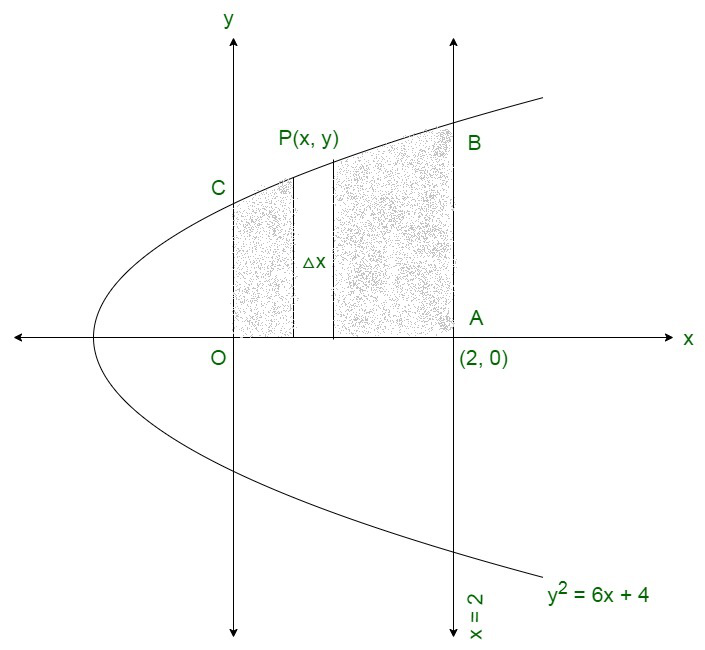Shaded region represents the required area that we have sliced in the form of a rectangle of

Width = △x

Length = y – 0 = y

Area of rectangle = y△x

This approximation rectangle slide from x = 0 to x = 2,

Required area = Region OABCORequired area =square units.

### Question 9. Draw the rough sketch of y2 + 1 = x, x ≤ 2. Find the area enclosed by the curve and the line x = 2.

Solution:

Here, we will find the area enclosed by x-axis

y2 = x + 1     ……….(1)

and

x = 2  ……………(2)

Equation (1) is a parabola with vertex at (0, 1) and axis as x-axis.

Equation (2) represents a line parallel to y-axis passing through  (2, 0)

Thus, here is the rough sketch;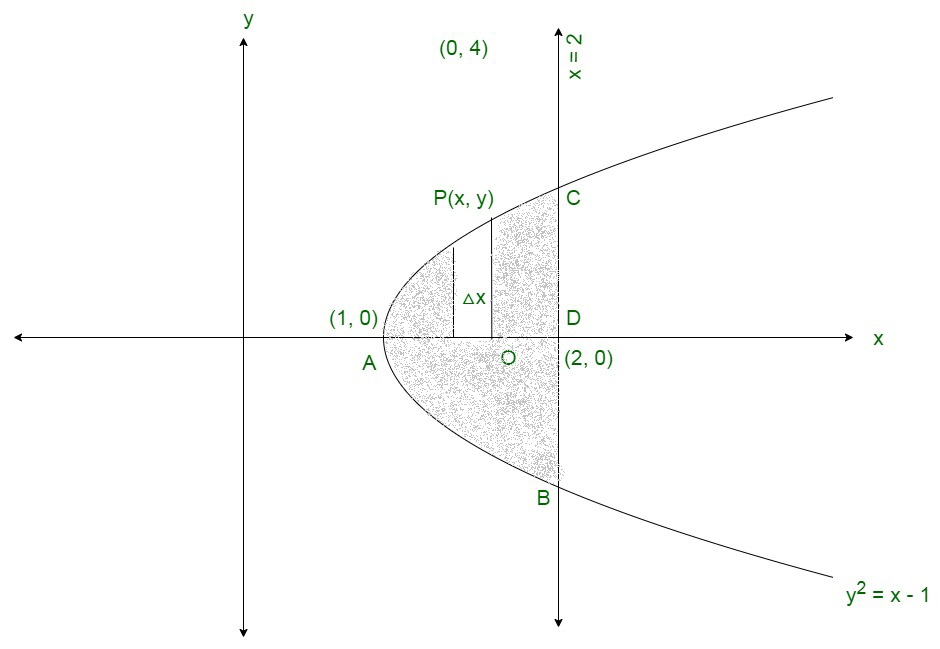Shaded region represents the required area that we have sliced in the form of a rectangle of

Width = △x

Length = y – 0 = y

Area of rectangle = y△x

This approximation rectangle slide from x = 0 to x = 2,

Required area = Region ABCA

= 2(Region AOCA)Required area =square units.

### Question 10. Draw a rough sketch of the graph of the curveand evaluate the area of the region under the curve and above the x-axis

Solution: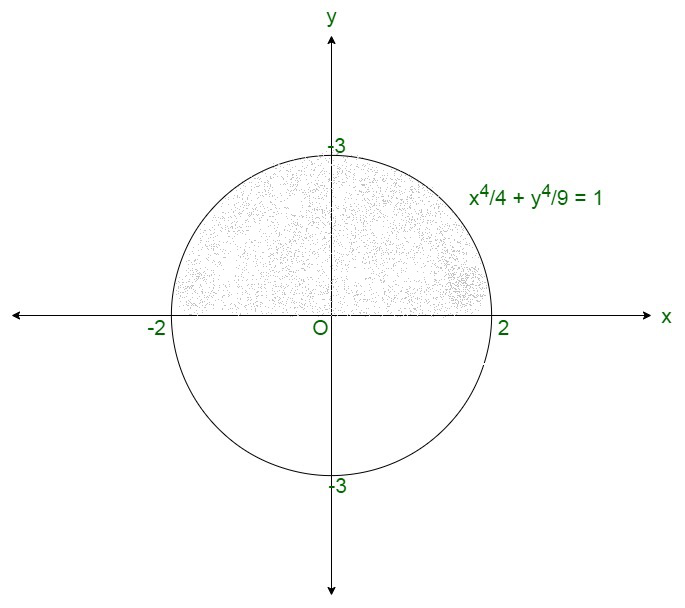Here, we can observe that ellipse is symmetrical about x-axis.

Area bounded by ellipse =My Personal Notes arrow_drop_up